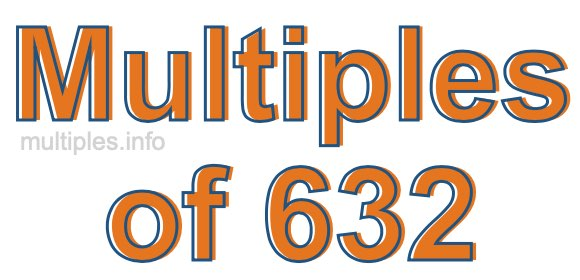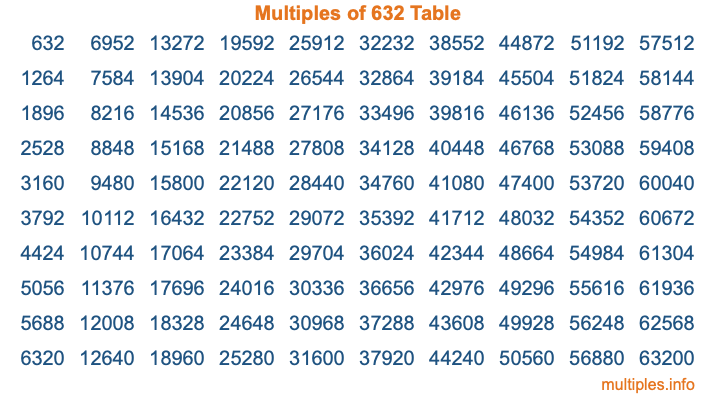Multiples of 632Welcome to the Multiples of 632 page. Here we will first teach you everything you will ever need to know about the multiples of 632, and then give you a study guide summary of everything we taught you to make sure you remember it all. Use this page to look up facts and learn information about the multiples of 632. This page will make you a multiples of six hundred thirty-two expert!

Definition of Multiples of 632
Multiples of 632 are all the numbers that when divided by 632 equal an integer. Each of the multiples of 632 are called a multiple. A multiple of 632 is created by multiplying 632 by an integer.

Therefore, to create a list of multiples of 632, you start with 1 multiplied by 632, then 2 multiplied by 632, then 3 multiplied by 632, and so on for as long as you want. Thus, the list of the first five multiples of 632 is 632, 1264, 1896, 2528, and 3160. To see a larger list of multiples of 632, see the printable image of Multiples of 632 further down on this page. We also have a category where you can choose any nth multiple of 632.

Multiples of 632 Checker
The Multiples of 632 Checker below checks to see if any number of your choice is a multiple of 632. In other words, it checks to see if there is any number (integer) that when multiplied by 632 will equal your number. To do that, we divide your number by 632. If the the quotient is an integer, then your number is a multiple of 632.

Is  a multiple of 632?

Least Common Multiple of 632 and ...
A Least Common Multiple (LCM) is the lowest multiple that two or more numbers have in common. This is also called the smallest common multiple or lowest common multiple and is useful to know when you are adding our subtracting fractions. Enter one or more numbers below (632 is already entered) to find the LCM.

Check out our LCM Calculator if you need more details about the Least Common Multiple or if you need the LCM for different numbers for adding and subtraction fractions.

nth Multiple of 632
As we stated above, 632 is the first multiple of 632, 1264 is the second multiple of 632, 1896 is the third multiple of 632, and so on. Enter a number below to find the nth multiple of 632.

th multiple of 632

Multiples of 632 vs Factors of 632
632 is a multiple of 632 and a factor of 632, but that is where the similarities end. All postive multiples of 632 are 632 or greater than 632. All positive factors of 632 are 632 or less than 632.

Below is the beginning list of multiples of 632 and the factors of 632 so you can compare:

Multiples of 632: 632, 1264, 1896, 2528, 3160, etc.

Factors of 632: 1, 2, 4, 8, 79, 158, 316, 632

As you can see, the multiples of 632 are all the numbers that you can divide by 632 to get a whole number. The factors of 632, on the other hand, are all the whole numbers that you can multiply by another whole number to get 632.

It's also interesting to note that if a number (x) is a factor of 632, then 632 will also be a multiple of that number (x).

Multiples of 632 vs Divisors of 632
The divisors of 632 are all the integers that 632 can be divided by evenly. Below is a list of the divisors of 632.

Divisors of 632: 1, 2, 4, 8, 79, 158, 316, 632

The interesting thing to note here is that if you take any multiple of 632 and divide it by a divisor of 632, you will see that the quotient is an integer.

Multiples of 632 Table
Below is an image of the first 100 multiples of 632 in a table. The table is in chronological order, column by column. The first column has the first ten multiples of 632, the second column has the next ten multiples of 632, and so on.The Multiples of 632 Table is also referred to as the 632 Times Table or Times Table of 632. You are welcome to print out our table for your studies.

Negative Multiples of 632
Although not often discussed or needed in math, it is worth mentioning that you can make a list of negative multiples of 632 by multiplying 632 by -1, then by -2, then by -3, and so on, to get the following list of negative multiples of 632:

-632, -1264, -1896, -2528, -3160, etc.

Multiples of 632 Summary
Below is a summary of important Multiples of 632 facts that we have discussed on this page. To retain the knowledge on this page, we recommend that you read through the summary and explain to yourself or a study partner why they hold true.

There are an infinite number of multiples of 632.

A multiple of 632 divided by 632 will equal a whole number.

632 divided by a factor of 632 equals a divisor of 632.

The nth multiple of 632 is n times 632.

The largest factor of 632 is equal to the first positive multiple of 632.

632 is a multiple of every factor of 632.

632 is a multiple of 632.

A multiple of 632 divided by a divisor of 632 equals an integer.

632 divided by a divisor of 632 equals a factor of 632.

Any integer times 632 will equal a multiple of 632.

Multiples of a Number
Here you can get the multiples of another number, all with the same attention to detail as we did for multiples of 632 on this page.

Multiples of
Multiples of 633
Did you find our page about multiples of six hundred thirty-two educational? Do you want more knowledge? Check out the multiples of the next number on our list!